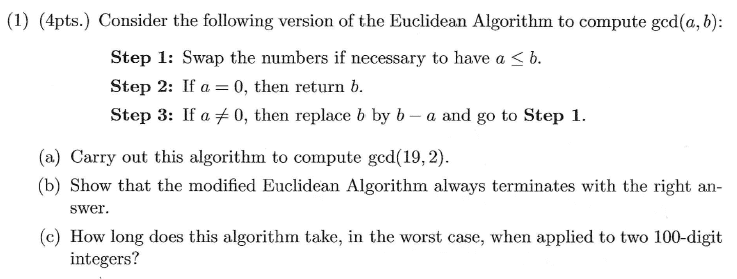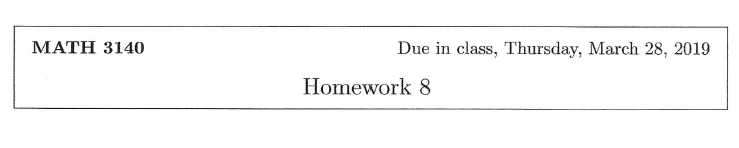# Modified Euclidean Algorithm proof

Robb
Homework Statement:
See attached problem 1 parts (b) and (c)
Relevant Equations:
b = ka + r (from part a)
gcd(f_n,f_{n-1})
gcd[f_{n-1},f_n - f_{n-1}]
gcd[(f_n - f_{n-1}), (f_{n-2} - f_{n-1})]
gcd[(f_{n-2} - f_{n-1}),f_{n-3} - f_{n-2})]
gcd[(f_{n-2} - f_{n-3}),(f_{n-4} - f_{n-3})]
.
.
.
gcd(f_2,f_1), where f_2 = 1, f_1 = 1

I assume LateX is not working yet. Not sure if I am on point here or not. Please advise. Thanks!

#### Attachments

• hw8.pdf
267.2 KB · Views: 181

Homework Helper
Dearly Missed
gcd(f_n,f_{n-1})
gcd[f_{n-1},f_n - f_{n-1}]
gcd[(f_n - f_{n-1}), (f_{n-2} - f_{n-1})]
gcd[(f_{n-2} - f_{n-1}),f_{n-3} - f_{n-2})]
gcd[(f_{n-2} - f_{n-3}),(f_{n-4} - f_{n-3})]
.
.
.
gcd(f_2,f_1), where f_2 = 1, f_1 = 1

I assume LateX is not working yet. Not sure if I am on point here or not. Please advise. Thanks!

Here is your submission done in PF LaTeX:
$$\begin{array}{l} \gcd(f_n,f_{n-1})\\ \gcd[f_{n-1},f_n - f_{n-1}]\\ \gcd[(f_n - f_{n-1}), (f_{n-2} - f_{n-1})]\\ \gcd[(f_{n-2} - f_{n-1}),f_{n-3} - f_{n-2})] \\ \gcd[(f_{n-2} - f_{n-3}),(f_{n-4} - f_{n-3})] \\ \vdots \\ \gcd(f_2,f_1), \text{where} f_2 = 1, f_1 = 1 \end{array}$$

It certainly works perfectly well. To make sure LaTeX knows when to start and end, use either "##" at the start and the end, to get an in-line formula or equation, or use "" (with no space between the two $s) at the start and the end to get a displayed equation. I used "$_nospace_\$" and got nicely-aligned results by inputting it all as an "array". Just right-click on the displayed output and ask for display of math as tex, to see the commands involved.

Robb
Shoot, I knew that but forgot. Thanks!

When you prove something, do specify the assumptions. Right now, the several rows of symbols you typed (tex or no tex) have close to zero meaning to anyone.

It is not the task of the person who is grading your work to translate it from scratch.

Under which assumptions do you proceed to the next line?
What is the meaning of the last line?!
Why does this algorithm terminate?
Why is the result correct?

•SammyS
Staff Emeritus
Homework Helper
Gold Member
I see that the new PF does not include the Problem Statement nor the Relevant Equations in the Quoted Portion when using the Reply feature. Here's a snip from the hw8.pdf document that you uploaded.And you need help with Problem 1 parts (b) and (c) .
Let's see an attempt.I suppose we (PF HW Helpers) should use our usual restraint when providing help, in case the due date is extended, or in case late work receives credit.

Robb
Homework was due 3/28. I received help from my instructor on part (a) of problem 1. Unfortunately, no late credit allowed.

The following is an attempt although it seams to me that the sequences that represent a & b converge to zero so I am confused. There is an assumption a>b for each line.

##(f_n,f_{n+1})## assume a>b
##(f_{n+1}, f_{n+1} - f_n)##
##(f_{n+1} - f_n,f_n - f_{n-1})##
##(f_n - f_{n-1},f_{n-2} - f_{n-1})##
##(f_{n-2} - f_{n-1},f_{n-3} - f_{n-2})##

Staff Emeritus
Homework Helper
Gold Member
Homework was due 3/28. I received help from my instructor on part (a) of problem 1. Unfortunately, no late credit allowed.

The following is an attempt although it seams to me that the sequences that represent a & b converge to zero so I am confused. There is an assumption a>b for each line.
Actually the requirement is that a ≤ b, and that's not so much an assumption as it is a step in the algorithm.​
##(f_n,f_{n+1})## assume a>b
##(f_{n+1}, f_{n+1} - f_n)##
##(f_{n+1} - f_n,f_n - f_{n-1})##
##(f_n - f_{n-1},f_{n-2} - f_{n-1})##
##(f_{n-2} - f_{n-1},f_{n-3} - f_{n-2})##
Whatever the ##f_i## refer to, I don't see how that makes sense.

See how the algorithm works for various situations. The example in part (a) is not all that enlightening, although it does show repeatedly subtracting 2 (a total of 9 times).
gcd(19, 2) Initially: a = 19, b = 2.​
Step 1: says to swap a and b.​
Now: a = 2 and b = 19 so b − a = 17.​
Now: a = 2 and b = 17 so b − a = 15.​
...
eventually you get to:​
Now: a = 2 and b = 5 so b − a = 3.​
Now: a = 2 and b = 3 so b − a = 1. So after the 9th subtraction of 2, we will have b < a .​
Now: a = 2 and b = 1 . We have now done integer division by repeated subtraction and found:​
19 divided by 2 has a quotient of 9 with a remainder of 1.​
Swap a and b. (The fact that we had b < a indicated that b = 1 was the remainder.)​
Now: a = 1 and b = 2 so b − a = 1.​
Now: a = 1 and b = 0 so Swap a and b, but note that the remainder is zero for 2 divided by 1.​
Now: a = 0 and b = 1 so: Return 1. This is gcd(19, 2) .​

Why not try the algorithm for some other specific cases:

gcd(19, 8), gcd(120, 84), gcd(42, 36), gcd(42, 30), gcd(12, 42), etc.